content

### Felix Gorbatsewich - The Ether and Universe

7. The attractive forces mechanism of physical bodies in the ethereal medium

As is known, sizes of atoms, including their electron shells are fractions and units of the unit of angstrom, Å = 1·10-10 m. The nuclei have the sizes close to 10-15 m. At the same time, the wavelength, for example of a visible light, is (4-7)·10-7 m . There are many experimental data about the propagation of light in gaseous, fluid and solid media.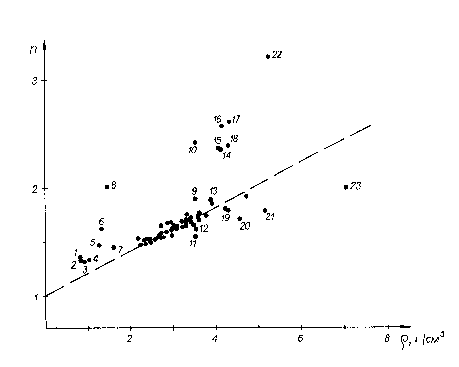Fig. 11. The relationship between the refraction coefficient n and the density r _ of some liquid and solid substances, minerals (based on the data [56, 58]). 1 - ice, 2 - acetone, 3 - alcohol, 4 - water, 5 - glycerine, 6 - carbon bisulphide, 7 - carbon tetrachloride, 8 - sulphur, 9 - titanite, 10 - diamond, 11 - grothite, 12 - topaz, 13 - siderite, 14 - wurtzite, 15 - sphalerite, 16 - brookite, 17 - rutile, 18 - goethite, 19 - xenotime, 20 - barite, 21 - monazite, 22 - hematite, 23 - cassiterite.

The refraction coefficient, which can be measured to a high accuracy in transparent media, is the parameter, closely related to the propagation velocity of a light wave. Let us consider a relationship between the refractive coefficient n and the density r of some substances (Fig. 11).

Parameters of the majority of substances (anhydrite, apatite, baddeleyite, beryl, boracite, galena, halite, gypsum, disthene, dolomite, calcite, quartz, cordierite, corundum, leucite, microcline, muscovite, nepheline, orthoclase, periclase, rhodonite, sillimanite, staurolite, zircon, eudialyte and lot of others) are subject to the relationship:

n = 1 + 0.2 r . (31)

This relationship has been reflected by a dotted line in Fig. 11. In the figure the substances, whose relations r and n are outside the general relation, are numbered. For example, the ratios for diamond, sulphur, iron, titanium and some of their compounds on the plot are above the line of the general relation. The relations for some compounds of fluorine, barium, phosphorous, tin, etc. are below this line.

As a whole, transparent substances, including gases, fluids and solid substances have the refraction coefficient n more than unity . It means that the light waves (photons) velocity in physical media is always lower, than in vacuum. It is natural to assume, that the deceleration of the velocity of light propagation in physical media happens due to the effect of bending around some, impenetrable for photons, areas. The photons of low energies have to bend the areas of space occupied by electron shells and atom nuclei. High-energy photons penetrate into the areas that are closer to a nucleus. X-ray waves interact directly with the area of an atomic nucleus. The deceleration of the light velocity of in physical media is contributed by the effects of photons re-emission, recombination and luminescence, to a greater degree. However, the basis, in which light waves propagate, is the ethereal medium. Thus, it is logical to assume, that the ethereal medium, being displaced by nuclear forces, is absent both close to an atomic nucleus and inside it.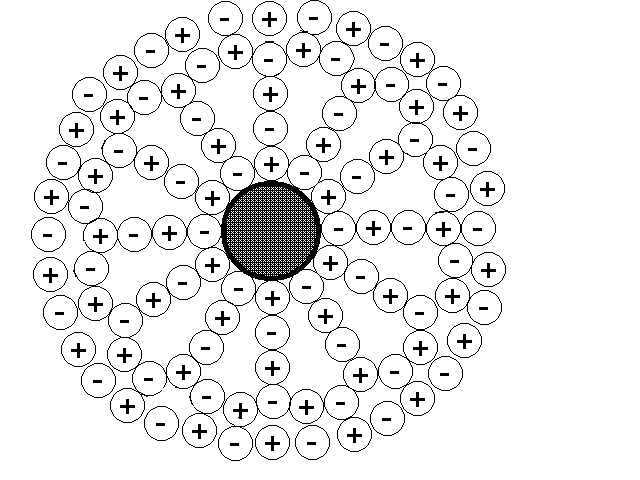Fig. 12. A simplified scheme of the spatial network structure of ether in the vicinity of a unit spherical mass.

In Figure 12 the structure of the ethereal medium near to a conditional atomic nucleus is represented as a unit spherical mass in simplified form. The given primitive scheme displays, that the spatial netlike structure of ether has been distorted by the spherical mass. Near to the spherical mass this structure is appreciably loosened. As the structure moves away from the spherical mass, the loosening degree will diminish. It is natural, that the sizes of such a mass, for example, an electron and a particle of the ethereal medium, are incomparable in sizes. The relation of their sizes is much greater than it is shown in Fig. 12.

A comparison of Fig. 8 and 12 shows that the structure, near to which there are no physical masses, has the greatest density. The structure distorted by mass presence, has a less density. A spatial netlike structure formed by unlike particles attracted to each other, develops great pressure on their contacts as was shown above.

The same or less pressure will be exerted on the spherical mass as well, Fig. 12. This pressure will be developed due to breaking contacts of unlike particles immediately contiguous to the spherical mass. The pressure on the spherical mass will be strengthened due to distortions of the second, third, fourth etc. line of the structural lattice, situated, accordingly, in the second, third, fourth etc. line from the spherical mass. This pressure is caused by a quest of particles situated in the second, third etc. line to be as close as possible to each other and to restore the non-deformation structure, Fig. 8.

At some greater distance from the centre of the spherical mass, the general view of the structural medium can be conventionally represented as concentric spheres inserted one into another, Fig. 13.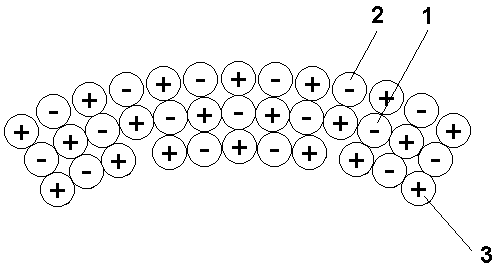Fig. 13. A fragment of the structure of the ethereal medium at some distance from a physical mass.

Conventionally, we shall consider, that in a medial concentric sphere (1, Fig. 13) all particles of the opposite kind contact with each other directly, without intervals. Then in the concentric sphere located further from the mass (2, Fig. 13), since the number of the opposite particles should correspond to each other, intervals will appear between them. In the concentric sphere located closer to a physical mass (3, Fig. 13), the package of particles will also be less dense, because it is impossible to dispose the same number of particles here, as in the medial sphere. Some of the particles from the near sphere will be forced out, and empty space will occupy their places.

The comparison of the diagrams, introduced in Fig. 8 and 13 enables to conclude that the ethereal medium in an environment of a physical mass is less dense and "looser", than in a medium without physical masses. It is easy to imagine that, as we move away from a physical mass, the density of the ethereal medium will increase, and its "loosening" will diminish proportionally to the distance from this mass.

If we imagine some physical test mass and place it inside a non-perturbed ethereal medium, Fig. 8, this test mass will distort the structure of the ethereal medium as it is shown in Fig. 12. The test mass will experience the greatest pressure, equal from all directions. Now let us move the test mass to the medium that has already been distorted by the presence of some physical mass, Fig. 13. In this case, the pressure on the test mass will not be identical from all directions. The test mass will be under pressure of a great many concentric layers of different curvature, depending on the distance to the physical mass. The concentric layers of lower curvature will exert the greater pressure on the trial mass. The pressure exerted by the layers with greater curvature that are closer to the physical mass, will be lower. Thus, the ethereal medium in the field of influence of a physical mass appears to be gradient. The vector of this gradient is directed to a physical body. The force pushing this body to a physical mass will be applied to the test body. This is just the fundamental basis for gravitational forces in the ethereal medium consisting of equal, opposite in sign particles.

Thus, a loose ethereal medium represents space, to which free masses from the area of space, with denser ethereal medium are displaced. If the lattice is curved, for example, due to the presence of some mass inside the lattice, it is less dense. In such a curved lattice, a free mass will move in the direction the lowering the gradient of the lattice density (or otherwise, in the direction of greater "loosening").

The law of gravitation is rather easily deduced from the above concepts. Let us assume, that along the circumference L1, of the concentric layer 1, Fig. 14, formed around of the heavy mass М1, the precise number n1 of particles of opposite signs with diameter d, or L1 = n1d, are stacked.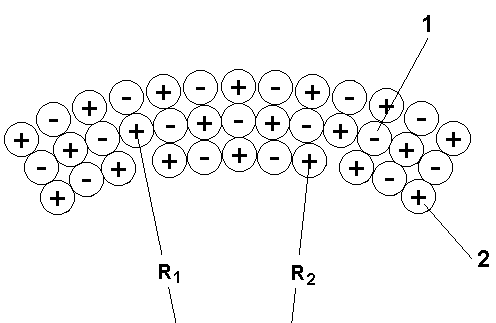Fig.14. The schema for quantity calculation of particles in concentric layers in the ethereal medium around a physical mass.

Let us consider, that L1 >> d. The radius of such a circle will be equal to R1 = n1d/2, and the quantity of particles n1 = 2p R1/d. As follows from our model the next concentric layer that is closer to the heavy mass with the circumference L2, will have the radius R2, smaller just by the magnitude of the particle d size than the first one, R2 = R1 - d. The circumference of layer 2 will be equal to L2 = 2p R2 = d(n1 - 2p ), and the number of particles n2 = 2p (R1 - d)/d. Otherwise, n2 = n1 - 2. Accordingly, in layer 2 the number of particles will be by 2p less than along the circumference L1. On the other hand, each particle of the circle L1 must be corresponded by another, opposite in sign, particle L2. Then due to the n - 2p number of particles in the second concentric layer 7 particles of the first layer will not be compensated. Therefore particles of layer 2 will be at slightly greater distance from each other, than the particles of the first layer. Thus, in the limits of concentric layer 2 some loosening of the ethereal medium occurs.

In some k-layer that is closer to the centre by the value kd, along the circumference the number of particles nk = n1 - 2kp will be stacked. The value of the ethereal medium loosening in the k-layer in relation to the first layer can be expressed by the coefficient, showing the ratio of the number of particles in each layer to their circles:

Dk = (n1 - 2кp)/n1 = 1 - 2кp/n1. (32)

The formula (32) in fact, at great numbers n, expresses a modification of the diameter (radius) or curvature of concentric layers, in the limits of which, ideally, the particles of ether are disposed.

It is easy to show, that with distance from the centre, the curvature (for spherical surfaces) diminishes proportionally to the square of the radius of the sphere. Accordingly, the degree of the vacuum medium "loosening" will decrease as much as the distance from the mass disturbing vacuum, will increase.

Let us imagine a presence of a point mass М1 in the homogeneous, non-perturbed vacuum. As was already shown, with distance from the point mass М1, the degree of vacuum "loosening" will diminish proportionally to the first power of the distance R to the centre of the mass that is М1/R. Now we shall introduce the second mass M2 into the point located at the distance R from the first mass. The mass M2 will cause "loosening" of the vacuum equal to М2/R at the area of the mass М1. Thus, the mutual attraction of the two masses М1 and М2 will be proportional to the product of two foregoing expressions,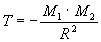. (33)

As is known, the law of gravitation is stated as follows: two material points possessing masses М1 and М2 are attracted to each other with the force F: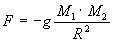, (34)

where R is the distance between the points, and g is the gravitation constant equal to 6.67× 10-11, n× m2/kg2 .

From this example it is clear, that the law of gravitation is directly deduced from the offered model of the ethereal medium. When analysing the formulas (33) and (34) one should take into consideration that ethereal particles are extremely small.

Thus, the presence of unit masses shown in Fig. 12, or their accumulation distorts the configuration of the spatial- netlike structure of the ethereal medium. As the unit masses concentrated mainly in nuclei are bound with each other by particular forces, forming solid, liquid and gaseous bodies, deformations introduced into the spatial-netlike structure, are partially summarized from each unit mass. In the end, it leads to loosening, lowering the specific density of the ethereal medium. The more is the total mass of a physical body, the greater is the loosening. It is great in the vicinity of planets. It is even greater in the vicinity of a massive star. Loosening of the ethereal medium created by galaxies, stretches for astronomical distances. The potential theory  allows one, from the given mass distribution, to determine mutual gravitational forces in planetary and more complicated systems.

The explanation of the nature of a mutual attraction of physical bodies, in our opinion is one of the most important results of the non-empty ether concept. As mentioned above, earlier I. Newton, MacGullagh, W. Thomson, et al. pointed to the presence of quasi-solid ether deformed by physical bodies [11, 12, 15, 32, 33]. There are experimental data confirming such a strain. For example, the light propagating in the environs of a massive body propagates with lower velocity than when it is far from it. During radiolocation of Mercury and Venus, as they moved behind the disk of the Sun, an additional signal delay stipulated by the gravitational field of our star, was about 2× 10-4 s . Thus, lowering of the rigidity, "loosening" and deformation of the ethereal medium near to physical bodies have been confirmed.

The proposed concept of the ethereal medium structure explains the nature of inertial forces, and the reason for identical acceleration of bodies of different mass in a gravitation field. Each physical body at rest occupies certain space in the ethereal medium, displacing a part of the netlike ether and distorting its structure, as it is shown in Fig. 12-14. Without the influence of gravitation masses, the ethereal medium will exert an equilateral pressure on this physical body. If a physical body moves uniformly, it will be flowed around by the ethereal medium. In the direction of the body's motion, in front of it, some mass of the ethereal medium will disconnect. Behind the body, the same mass, with the same velocity, as in front of the body, will close. Moment of momentum of masses located along the line of motion in front of the body and behind it will be equal. As the ethereal medium has no ability to absorb, disperse energy, uniform motion of a physical body can continue indefinitely long.

Another situation will be observed during acceleration of a physical body motion. In this case moment of momentum of the ethereal medium mass located in front of the body and behind it will differ. To cause an acceleration of a physical body it is necessary, according to the second Newton's law, to apply force to it. To cause an acceleration of a heavier body, it is necessary to disconnect a much greater number of particles of the ethereal medium proportional to the mass of this body along the line of its motion. Thus, the acceleration of a light and heavy body, for example, in the gravitation field of the Earth, will be identical. The absence of ether, as a medium, actively interacting with an accelerated mass, contradicts the third Newton's law of action and reaction.

It is the interaction of the accelerating charge with the ether medium that enabled J.J. Larmor  to propose the following hypothesis: since atoms consist of electron systems, it may be proved that the inertia of ordinary weighty matter can be explained by the excitation of self-inductance currents during acceleration of the weighty body. Every electron, as it accelerates together with the body, excites convection self-inductance currents around itself. In this case, according to the energy conservation law, some work should be done to produce these currents (local magnetic field of self-inductance) and to set the electron in motion.

But this hypothesis was not supported by physicists at that time. To our mind, the idea of J.J. Larmor is fruitful and it is necessary to return to its development.

A physical body moving uniformly in the ether medium does not meet resistance. Even D’Alambere pointed to the possibility of a body to maintain a strait-line uniform motion meeting no resistance in an ideal liquid. Thus, the ether medium resembles an ideal liquid by its properties, but at the same time it has some distinctions of a specific solid body.

content